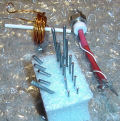# Estimating Kinetic Energy

We want to know the expected kinetic energy of the projectile to estimate the velocity of various projectiles.

## Kinetic EnergyNote that potential energy PE = CV2/2 (where C is farads, and V is voltage)

Also note kinetic energy KE = mv2/2 (where m is kilograms and v is meters/sec)

In coilguns, KE is related to PE by an efficiency factor 'e' defined as: e = (KE)/(PE)

Set the kinetic energy equal to the efficiency multiplied by potential energy, and solve for velocity:Assuming typical values for this coilgun:

• e = 0.01 - 0.02 (e.g. 1 - 2%) based on experience at lower energies
• C = 12,000 uF
• V = 100 - 190 volts
• m = 0.02163 or 0.04301 kg (2 to 4 grams, e.g. mass of some known finishing nails
• Note: 1 m/s = 3.281 fps

At minimum speed:

• e = 0.01, C = 0.012 F, m = 0.04301 kg, V = 100v, which yields v = 5.2 m/s = 17.1 fps

At maximum speed:

• e = 0.02, C = 0.012 F = 0.02163 kg, V = 190v, which yields v = 20.0 m/s = 65.7 fps
 < Previous Page 6 of 37 Next >